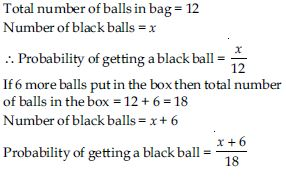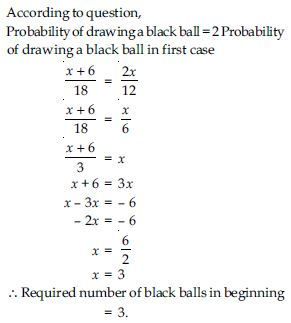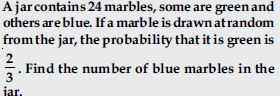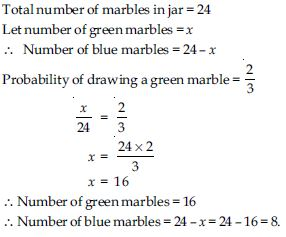# NCERT Solutions for Class 10 Math Chapter 15 - Probability

For effective and efficient exam preparation, NCERT Solutions for Class 10th at Aasoka is the best study material. Grab free educational resources to kick start your learning process. These solutions are accurately designed by the team of professionals keeping in the mind the updated syllabus for Class 10th Math. Only those topic questions are included which is coming in the board exam. So, studying from NCERT Solutions will help students in covering the entire syllabus. With good preparation and regular practice, scoring good grades is not a difficult task for students.

Class 10th Math Chapter 15 will teach “Probability”. Students will get to learn the difference between theoretical probability and experimental probability, elementary events, finding the probability of different events, etc. With every topic, several examples are provided in the chapter so that students can efficiently understand the concept better.

##### Question 1:

Probability of an event E + Probability of the event ‘not E’ = _____.

1

##### Question 2:

The probability of an event that cannot happen is _____. Such an event is called _____.

0, impossible event

##### Question 3:

The probability of an event that is certain to happen is ____________. Such an event is called __________.

1, sure event

##### Question 4:

The sum of the probabilities of all the elementary events of an experiment is ____.

1

##### Question 5:

The probability of an event is greater than or equal to _____ and less than or equal to _____.

0, 1

##### Question 6:

Which of the following experiments have equally likely outcomes? Explain
A driver attempts to start a car. The car starts or does not start.

When a driver attempts to start a car the car starts normally. Only when there is some defect, the car does not start. So, the outcome is not equally likely.

##### Question 7:

Which of the following experiments have equally likely outcomes? Explain
A player attempts to shoot a basketball. She/he shoots or misses the shot.

When a player attempts to shoot a basketball the outcome in this situation is not equally likely because the outcome depends on many factors: Such as; the training of the player, height of the player etc.

##### Question 8:

Which of the following experiments have equally likely outcomes? Explain

Since, for a question there are two possibilities either right or wrong the outcome in this trial of true-false question is either true or false i.e. one out of the two and both have equal chances to happen. Hence, the two outcomes are equally likely.

##### Question 9:

Which of the following experiments have equally likely outcomes? Explain
A baby is born. It is a boy or a girl.

A new baby (i.e. who took birth at a moment) can be either a boy or a girl and both the outcomes have equally likely chances.

##### Question 10:

Why is tossing a coin considered to be a fair way of deciding which team should get the ball at the beginning of a football game?

When a coin is tossed there are only two possibilities i.e. head or tail both are equally likely to happen. Result of the toss of a fair coin is completely unpredictable.

##### Question 11: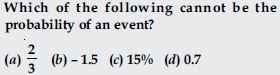As we know probability of an event cannot be less than 0 and greater than 1.
i.e. 0 $\le$ P $\le$ 1
∴ (b) – 1.5 is not possible.

##### Question 12:

If P(E) = 0.05, what is the probability of ‘not E’?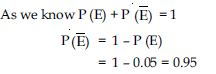##### Question 13:

A bag contains lemon flavoured candies only. Malini takes out one candy without looking into the bag. What is the probability that she takes out
an orange flavoured candy?

Since, bag contains only lemon flavoured candies
∴ There is no orange candy,
∴ It is an impossible event.
∴ Probability of getting an orange flavoured
candy = 0

##### Question 14:

A bag contains lemon flavoured candies only. Malini takes out one candy without looking into the bag. What is the probability that she takes out
a lemon flavoured candy?

Since, there are only lemon flavoured candies, it is a sure event.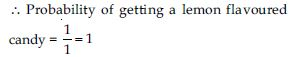##### Question 15:

It is given that in a group of 3 students, the probability of 2 students not having the same birthday is 0.992. What is the probability that the 2 students have the same birthday?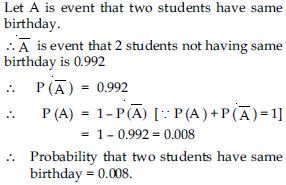##### Question 16:

A bag contains 3 red balls and 5 black balls. A ball is drawn at random from the bag. What is the probability that the ball drawn is (i) red? (ii) not red?

Number of red balls = 3
Number of black balls = 5
Total number of balls = 3 + 5 = 8
One ball is drawn at random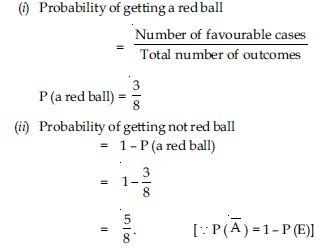##### Question 17:

A box contains 5 red marbles, 8 white marbles and 4 green marbles. One marble is taken out of the box at random. What is the probability that the marble taken out will be (i) red? (ii) white? (iii) not green?

Number of red marbles = 5
Number of white marbles = 8
Number of green marbles = 4
Total number of marbles = 5 + 8 + 4 = 17
Since, one marble is taken out.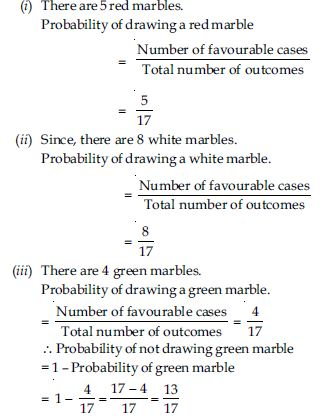##### Question 18:

A piggy bank contains hundred 50p coins, fifty ₹1 coins, twenty ₹2 coins and ten ₹5 coins. If it is equally likely that one of the coins will fall out when the bank is turned upside down, what is the probability that the coin (i) will be a 50p coin? (ii) will not be a ₹5 coin?

Number of 50p coins = 100
Number of ₹1 coins = 50
Number of ₹2 coins = 20
Number of ₹5 coins = 10
∴Total number of coins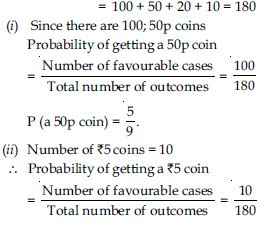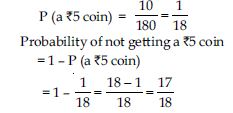##### Question 19:

Gopi buys a fish from a shop for his aquarium. The shopkeeper takes out one fish at random from a tank containing 5 male fish and 8 female fish. What is the probability that the fish taken out is a male fish?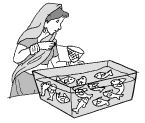Number of male fish = 5
Number of female fish = 8
Total number of fish in the tank = 5 + 8 = 13
Probability of getting a male fish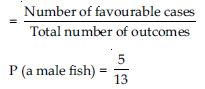##### Question 20:

A game of chance consists of spinning an arrow which comes to rest pointing at one of the numbers 1, 2, 3, 4, 5, 6, 7, 8 and these are equally likely outcomes. What is the probability that it will point at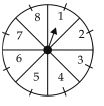8?

Total number of outcomes
= {1, 2, 3, 4, 5, 6, 7, 8} = 8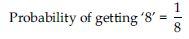##### Question 21:

A game of chance consists of spinning an arrow which comes to rest pointing at one of the numbers 1, 2, 3, 4, 5, 6, 7, 8 and these are equally likely outcomes. What is the probability that it will point atan odd number?

Odd numbers are = {1, 3, 5, 7} = 4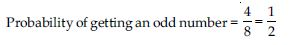##### Question 22:

A game of chance consists of spinning an arrow which comes to rest pointing at one of the numbers 1, 2, 3, 4, 5, 6, 7, 8 and these are equally likely outcomes. What is the probability that it will point ata number greater than 2?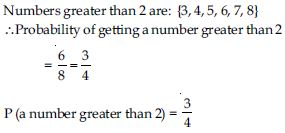##### Question 23:

A game of chance consists of spinning an arrow which comes to rest pointing at one of the numbers 1, 2, 3, 4, 5, 6, 7, 8 and these are equally likely outcomes. What is the probability that it will point ata number less than 9?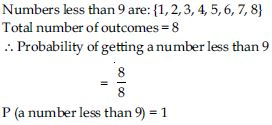##### Question 24:

A die is thrown once. Find the probability of getting
a prime number

When dice is thrown number of possible outcomes
S = {1, 2, 3, 4, 5, 6} = 6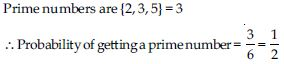##### Question 25:

A die is thrown once. Find the probability of getting
a number lying between 2 and 6

When dice is thrown number of possible outcomes
S = {1, 2, 3, 4, 5, 6} = 6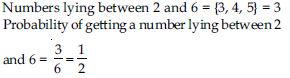##### Question 26:

A die is thrown once. Find the probability of getting
an odd number.

When dice is thrown number of possible outcomes
S = {1, 2, 3, 4, 5, 6} = 6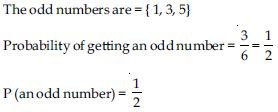##### Question 27:

One card is drawn from a well-shuffled deck of 52 cards. Find the probability of getting
a king of red colour

There are 52 cards in a pack. There are two red kings i.e. king of heart and king of diamond.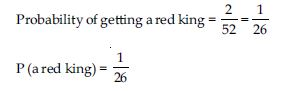##### Question 28:

One card is drawn from a well-shuffled deck of 52 cards. Find the probability of getting
a face card

There are 52 cards in a pack.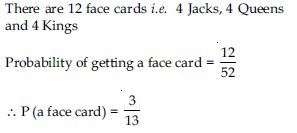##### Question 29:

One card is drawn from a well-shuffled deck of 52 cards. Find the probability of getting
a red face card

There are 52 cards in a pack.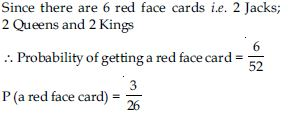##### Question 30:

One card is drawn from a well-shuffled deck of 52 cards. Find the probability of getting
the jack of hearts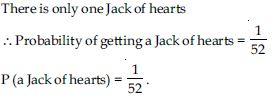##### Question 31:

One card is drawn from a well-shuffled deck of 52 cards. Find the probability of getting

There are 52 cards in a pack.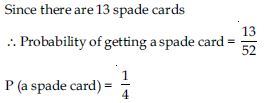##### Question 32:

One card is drawn from a well-shuffled deck of 52 cards. Find the probability of getting
the queen of diamonds

There are 52 cards in a pack.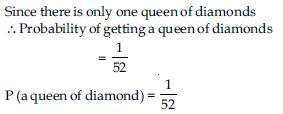##### Question 33:

Five cards – the ten, jack, queen, king and ace of diamonds, are well-shuffled with their face downwards. One card is then picked up at random.
What is the probability that the card is the queen?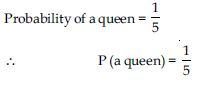##### Question 34:

Five cards – the ten, jack, queen, king and ace of diamonds, are well-shuffled with their face downwards. One card is then picked up at random.
If the queen is drawn and put aside, what is the probability that the second card picked up is (a) an ace? (b) a queen?

Five cards are ten, jack, queen, king and ace.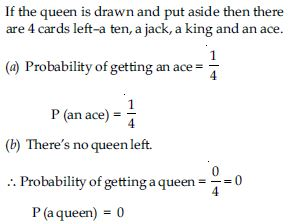##### Question 35:

12 defective pens are accidentally mixed with 132 good ones. It is not possible to just look at a pen and tell whether or not it is defective. One pen is taken out at random from this lot. Determine the probability that the pen taken out is a good one.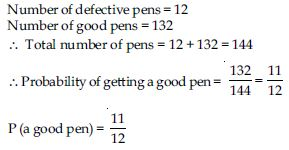##### Question 36:

A lot of 20 bulbs contains 4 defective ones. One bulb is drawn at random from the lot. What is the probability that this bulb is defective?

Number of defective bulbs = 4
Number of good bulbs (not defective) = 16
Total number of bulbs = 4 + 16 = 20
Probability of getting a defective bulb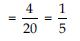##### Question 37:

A lot of 20 bulbs contains 4 defective ones. One bulb is drawn at random from the lot. Suppose the bulb drawn is not defective and is not replaced. Now one bulb is drawn at random from the rest. What is the probability that this bulb is not defective?

When a defective bulb drawn is not being replaced, we are left with 19 bulbs.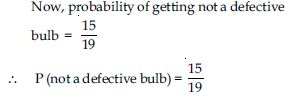##### Question 38:

A box contains 90 discs which are numbered from 1 to 90. If one disc is drawn at random from the box, find the probability that it bears
a two-digit number

From 1 to 90 there are 90 numbers in all and 81 two-digit numbers from 10 to 90.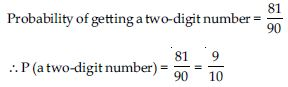##### Question 39:

A box contains 90 discs which are numbered from 1 to 90. If one disc is drawn at random from the box, find the probability that it bears
a perfect square number

From 1 to 90 there are 90 numbers in all and 81 two-digit numbers from 10 to 90.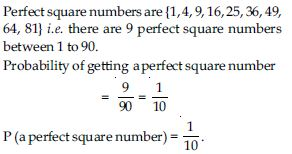##### Question 40:

A box contains 90 discs which are numbered from 1 to 90. If one disc is drawn at random from the box, find the probability that it bears
a number divisible by 5.

From 1 to 90 there are 90 numbers in all and 81 two-digit numbers from 10 to 90.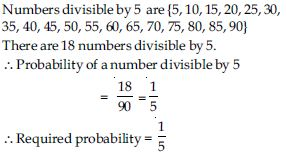##### Question 41:

A child has a die whose six faces show the letters as given below: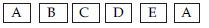The die is thrown. What is the probability of getting (i) A? (ii) D?

Number of faces of a die = 6
S = {A, B, C, D, E, A}
n (S) = 6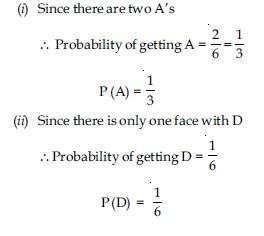##### Question 42:

Suppose you drop a die at random on the rectangular region shown in the figure. What is the probability that it will land inside the circle with diameter 1 m?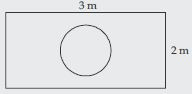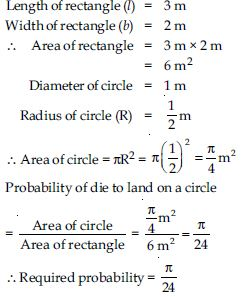##### Question 43:

A lot consists of 144 ball pens of which 20 are defective and the others are good. Nuri will buy a pen if it is good, but will not buy it if it is defective. The shopkeeper draws one pen at random and gives it to her. What is the probability that

Total number of pens in lot = 144
Number of defective pens = 20
∴ Number of good pens = 144 – 20 = 124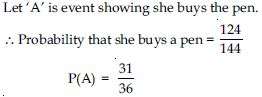##### Question 44:

A lot consists of 144 ball pens of which 20 are defective and the others are good. Nuri will buy a pen if it is good, but will not buy it if it is defective. The shopkeeper draws one pen at random and gives it to her. What is the probability that

Total number of pens in lot = 144
Number of defective pens = 20
∴ Number of good pens = 144 – 20 = 124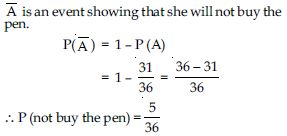##### Question 45:

Two dice, one blue and one grey are thrown at the same time. Write down all the possible outcomes.
Complete the following table: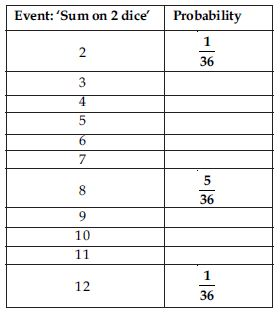When two dice are thrown, total number of possible outcomes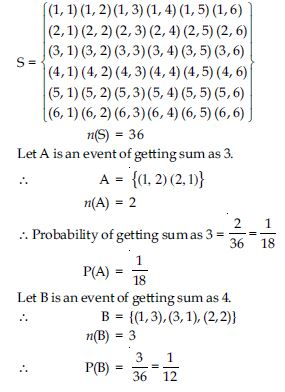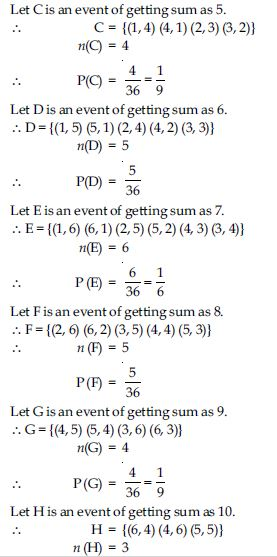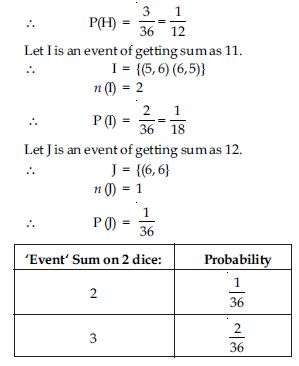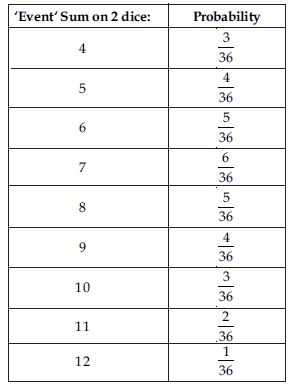##### Question 46:

Two dice, one blue and one grey are thrown at the same time. Write down all the possible outcomes.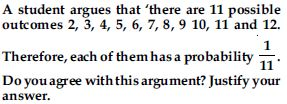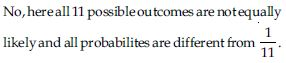##### Question 47:

A game consists of tossing a one rupee coin 3 times and noting its outcome each time. Hanif wins if all the tosses give the same result i.e., three heads or three tails, and loses otherwise. Calculate the probability that Hanif will lose the game.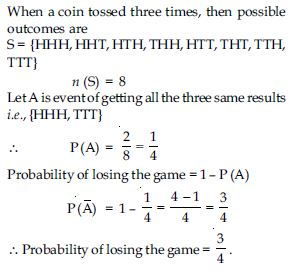##### Question 48:

A die is thrown twice. What is the probability that
(i) 5 will not come up either time?
(ii) 5 will come up at least once?

When a die is thrown twice all possible outcomes are: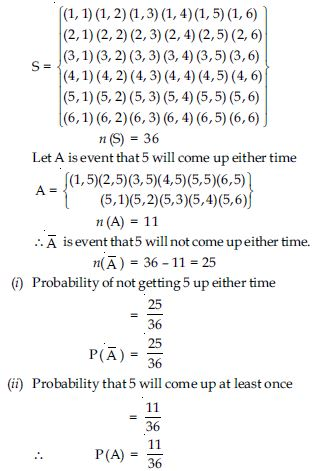##### Question 49:

Which of the following arguments are correct and which are not correct? Give reasons for your answer: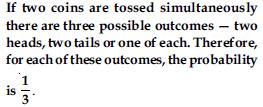When two coins are tossed the possible outcomes are S = {HH, HT, TH, TT}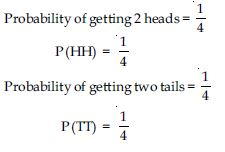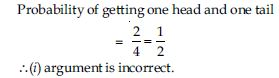##### Question 50:

Which of the following arguments are correct and which are not correct? Give reasons for your answer: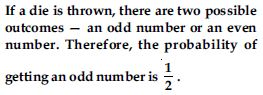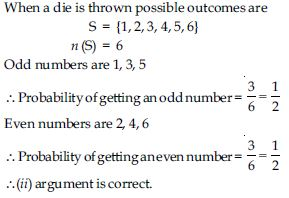##### Question 51:

Two customers Shyam and Ekta are visiting a particular shop in the same week (Tuesday to Saturday). Each is equally likely to visit the shop on any day as on another day. What is the probability that both will visit the shop on (i) the same day? (ii) consecutive days? (iii) different days?

When Shyam and Ekta visiting a particular shop in the same week. Possible outcomes are: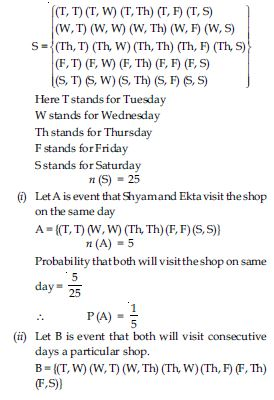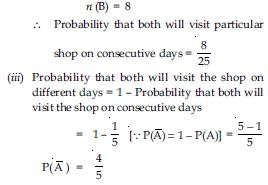##### Question 52:

A die is numbered in such a way that its faces show the numbers 1, 2, 2, 3, 3, 6. It is thrown two times and the total score in two throws is noted. Complete the following table which gives a few values of the total score on the two throws: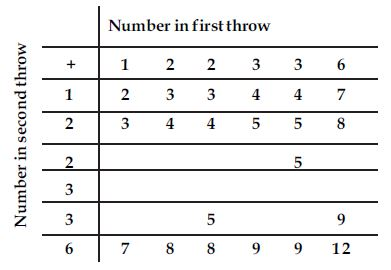What is the probability that the total score is
even?

The complete table is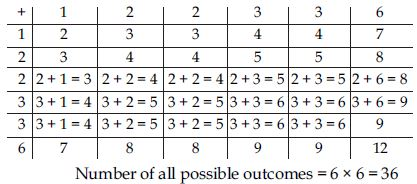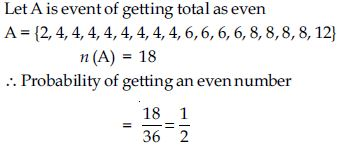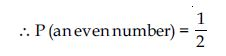##### Question 53:

A die is numbered in such a way that its faces show the numbers 1, 2, 2, 3, 3, 6. It is thrown two times and the total score in two throws is noted. Complete the following table which gives a few values of the total score on the two throws:What is the probability that the total score is
6?

The complete table is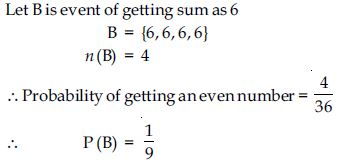##### Question 54:

A die is numbered in such a way that its faces show the numbers 1, 2, 2, 3, 3, 6. It is thrown two times and the total score in two throws is noted. Complete the following table which gives a few values of the total score on the two throws:What is the probability that the total score is
at least 6?

The complete table is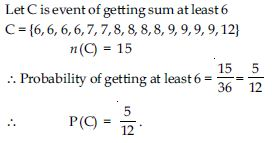##### Question 55:

A bag contains 5 red balls and some blue balls. If the probability of drawing a blue ball is double that of a red ball, determine the number of blue balls in the bag.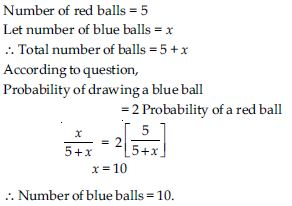##### Question 56:

A box contains 12 balls out of which x are black. If one ball is drawn at random from the box, what is the probability that it will be a black ball? If 6 more black balls are put in the box, the probability of drawing a black ball is now double of what it was before. Find x.